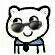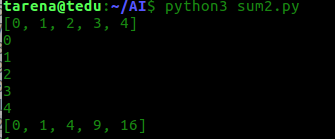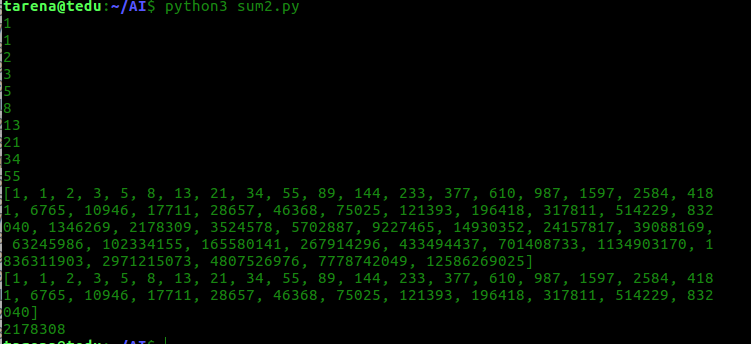#Python全栈工程师（多继承、函数重写）

0
0
01. 云栖社区>
2. 博客>
3. 正文

## Python全栈工程师（多继承、函数重写）

ParisGabriel

每天坚持手写  一天一篇  决定坚持几年 为了梦想为了信仰开局一张图

Python人工智能从入门到精通

对象 ------------------------->

单继承：
一个子类只有一个父类
一个父类可以有多个子类

--------------------------------------------------------------------------------------------

issublclass（cls， cls_or_tuple）
判断这个类是否继承自其他类， 如果此cls是class
或tuple中的一个派生子类， 返回True 否则返回False

class A:
pass
class B(A):
pass
class C(B):
pass

issublclass(C, B) # True
issublclass(B, C) # False
issublclass(C, (int, str)) # False
issublclass(C, (int, B, str)) # True

封装是指隐藏类实现细节让使用者不关心这些细节
封装的目的是让使用者通过尽可能少的方法（或属性）操作对象

python类中以双下划线（__）开头
双下划线结尾的标识符为私有成员，私有成员或只能用类的方法进行访问和修改
以__开头的实例变量有私有属性
以__开头的方法有私有方法

```class A:
def __init__(self):
self.__p1 = 100  # 私有属性

def show_A(self):
print("self.__p1", self.__p1)
self.__m1()  # 可以调用自己的方法

def __m1(self):  # 私有方法
print("__m1(self)方法被调用")

a = A()
a.show_A()  # 100
# print(a.__p1)  # 出错　不能在类外部访问a.__p1私有属性　也不能在子类中访问
# a.__m1()  # 出错　不能在类外部访问a.__p1私有方法　也不能在子类中访问

class B(A):
pass

b = B()
print(b.__p1)  # 出错，子类不能访问父类的私有成员
b.__m1()  # 出错

b._A__p1 = 200  # python的假封装可以用此方法访问
print(b.__p1)  # 200```

什么是多态
就是多种状态
多态是指在继承/派生关系的类中，调用基类对象的方法，
实际能够调用子类的覆盖方法的现象叫多态

静态（编译时状态）执行速度快
动态（运行时状态）执行速度慢

多态调用方法与对象相关不与类相关
Python的全部对象只有“运行时状态（动态）”
没有C++语言里的“编译时状态（静态）”
由于Python是解释执行的 是动态 没有静态
是在运行时编译（解释执行）

```# Pytgon中的运行时状态

class Shape:
def draw(self):
print("Shape的　draw　方法被调用")

class Point(Shape):
def draw(self):
print("画车")

class Circle(Shape):
def draw(self):
print("画圈")

# python无法实现静态　除非不出现任何覆盖
def my_draw(s):  # 其他静态语言　def　my_draw(Circle s)　指定调用者　无法进行改变
s.draw()  # 此处显示出＂动态＂

s1 = Circle()
s2 = Point()
my_draw(s1)  # 只有在调用时才能能确定调用哪一个
my_draw(s2)```

继承
封装
多态

语法：
class 类名（基类名1， 基类名2， ...）
说明：
1.一个子类同时继承自多个父类，父类中的方法可以同时被继承下来
2.如果两个父类中同时有同名方法，而在子类中又没有覆盖此方法时，
调用结果难以确定  （也可以确定 C3算法）

```class Car:
def run(self, speed):
print("汽车", speed, "Km/h 的速度行驶")

class Plane:
def fly(self, height):
print("飞机以海拔", height, "米高度飞行")

class PlaneCar(Plane, Car):
'''同时继承Plane,Car的方法'''

p1 = PlaneCar()
p1.fly(10000)
p1.run(300)```

标识符冲突的问题
要谨慎使用继承

```# 多继承名字冲突问题

class A:
def m(self):
print("A.m被调用")

class B:
def m(self):
print("B.m被调用")

class AB(A, B):  # 优先调用先出现的父类　　有先后顺序
pass

ab = AB()

ab.m()  # A.m被调用

class BA(B, A):
pass

ba = BA()

ba.m()  # B.m被调用```

类的__mro__属性
__mro__ 一个元组 里面存放类
此属性用来记录方法查找顺序

```class A:
def go(self):
print("A")

class B(A):
def go(self):
print("B")

class C(A):
def go(self):
print("C")

class D(B, C):
def go(self):
print("D")
super().go()

d = D()
d.go()  # B

# 多继承的super调用关系一定是mro元组内的下一个类　和子父类没关系
# 如果没有方法则报错　　调用算法（C3算法）
# 正常调用也是mro顺序```

在自定义类内添加相应的方法自定义类创建的实例
像内建对象一样进行内建函数操作

repr（obj） 返回一个能代表此对象的表达式字符串，通常eval（repr（obj））== obj
（这个字符串通常给Python解释执行器运行用的）
str（obj） 返回字符串（这个字符串通常给人阅读用的）

repr（obj） 函数的重写方法：
def __repr__（self）：

pass
str（obj） 函数的重写方法：
def __str__（self）：

pass
说明：
1.str（obj）函数先查找obj.str（）方法
调用此方法并返回结果
2.如果没有obj.__str__方法时返回obj.__repr__（）
方法的结果并返回
3.如果obj.__repr__方法不存在，则调用obj类的__repr__
实例方法显示：<__main__.XXXX object at 0x7f4b1c36fa90>

```class MyNumber:
def __init__(self, value):
'构造函数,初始化MyNumber'
self.data = value

def __str__(self):
'''转换为人能够识别的字符串'''
print("__str__方法被调用")
return "自定义数字类型对象：%d" % self.data

def __repr__(self):
'''转换为eval能够识别的字符串'''
return 'MyNumber(%d)' % self.data

n1 = MyNumber(100)
n2 = MyNumber(200)
print("repr(n1):--->", repr(n1))
print("strr(n2):--->", str(n2))
print("strr(n2):--->", n2.__str__())```

__abs__ 　　　　   abs（obj） 　　　　 　   函数
__len__ 　　　　   len(obj) 　　　　　　　  函数（必须返回整数）
__reversed__　　  reversed(obj) 　　 函数（必须返回可迭代对象）
__round__ 　　      round(obj) 　　　　   函数

```# 函数重写
class MyNumber:

def __init__(self, v):
self.data = v

def __repr__(self):
return 'MyNumber(%d)' % self.data

def __abs__(self):
'''__abs__函数重写 求绝对值函数'''
# return -self.data
v = abs(self.data)
return MyNumber(v)  # 创建一个新的MyNumber对象

def __len__(self):
'''__len__函数重写 求长度函数'''
# return len(self.data)
i = 0
for x in self.data:
i += 1
return i

i1 = MyNumber(-10)
print(i1)
i2 = abs(i1)
print(i2)

i3 = MyNumber("123d5sa")
print(len(i3))```

__complex__ 　　  complex(obj) 　   　函数
__int__ 　　　   　 int(obj) 　　　　　  函数
__float__ 　　　　 float(obj) 　　　　  函数
__bool__ 　　　　 bool(obj) 　　　　  函数

```数据转换构造函数重写
class MyNumber:

def __init__(self, v):
self.data = v

def __repr__(self):
return 'MyNumber(%d)' % self.data

def __int__(self):
# return 999999  # 可以自定义返回规则
return self.data

n1 = MyNumber(100)
x = int(n1)
print(type(x))  # <class 'int'>

print(bool(n1))  # True
n2 = MyNumber(0)
print(bool(n2))  # True

# 默认返回  # True```

格式
__bool__
作用：
用于bool（obj）函数取值
用于if语句的真值表达式中
说明：
1.当自定义的类内有__bool__(self)
方法时，此方法的返回值为bool（obj）的返回值
2.当不存在__bool__(self)，bool（x）
返回__len__（self）方法的返回值是否为零测试布尔值
3.当不存在__len__方法时，则直接返回True

```# bool(x)函数重写
class MyList:
'''自定义类型的列表，用来保存数据，内部用列表来储存数据'''
def __init__(self, iterable):
self.data = [x for x in iterable]

def __repr__(self):
return " MyList(%s)" % self.data

def __len__(self):
'''返回长度'''
print("__bool__方法被调用")
return len(self.data)

# def __bool__(self):
#     print("__bool__方法被调用")
#     return False  # 此处定义所有对象返回False

def __bool__(self):
print("__bool__方法被调用")
for x in self.data:
if not x:
return False
return True  # 自定义返回规则（这就是函数重写）

myl = MyList((1, 2, -3, 4, -5, 5))
print(myl)
print(bool(myl))
if myl:
print("myl是真值")
else:
print("myl是假值")```

什么是迭代器：
可以通过next（it）函数取值的对象就是迭代器
迭代器协议：
迭代器对象能够使用next函数获取下一项数据
在没有下一项数据时触发一个StopIteration异常终止迭代的重写

__next__（self） 方法来实现迭代协议

class MyIterator：
def __next__(self):
迭代器协议
return 数据

是指 iter（obj） 函数返回迭代对象（实例）
可迭代对象内部需要定义__iter__（self）方法来返回迭代器对象

```# 此示例示意让自定义的作为可迭代对象能让 for 语句迭代访问
class MyList:
'''创建MyList类'''
def __init__(self, iterable=()):
'''初始化self
iterable:可迭代对象
'''
# 用iterable生成列表
self.data = [x for x in iterable]

def __repr__(self):
'''返回一个MyList字符串'''
return 'MyList(%s)' % self.data

def __iter__(self):
'''此方法必须返回一个迭代器对象
此方法创建一个迭代器对象并返回
'''
return MyListIterator(self.data)

class MyListIterator:
'''此类用来创建迭代器,此类型的迭代器可以迭代访问
MyList类型的对象'''
def __init__(self, lst):
self.data = lst
self.cur_index = 0  # 初始化迭代器的起始位置

def __next__(self):
'''此方法用于实现迭代器协议'''
if self.cur_index >= len(self.data):
# 如果索引越界就发终止迭代通知
raise StopIteration
value = self.data[self.cur_index]  # 要返回的值
self.cur_index += 1
return value

myl = MyList([0, -1, 2, -3])
print(myl)

# it = iter(myl)
# print(next(it))

for x in myl:  # 迭代访问自定义类型的可迭代对象
print(x)```

class Bicycle:
def run(self, km):
print('自行车骑行了', km, '公里')

1. fill_charge(self, vol) 用来充电, vol为电量
2. run(self, km) 方法每骑行10km消耗电量1度,同时显示当前电量,当电量耗尽时调用 父类的run方法继续骑行

b = EBicycle(5) # 新买的电动有内有5度电
b.run(10) # 电动骑行了10km还剩 4 度电
b.run(100) #电动骑行了40km,还剩0度电,其余60用脚登骑行
b.fill_charge(10) # 又充了10度电
b.run(50) # 骑行了50公里剩5度电

```class Bycycle:
def run(self, km):
'''自行车'''
print("自行车骑行了", km, "公里")

class EBycyle(Bycycle):
def __init__(self, volume=0):
'''初始化电动车'''
self.volume = volume
print("新买的电动车有", volume, "度电")

def fill_charge(self, vol):
'''冲电'''
self.volume += vol
print("又冲了", vol, "度电")

def run(self, km):
self.volume -= (km / 10)  # 减去使用的电量
x = abs(self.volume) * 10  # 计算超出的公里
if self.volume < 0:  # 判断是否超出电量
self.volume = 0
print("电动车骑行了", km, "千米　还剩",
self.volume, "度电 其余", x, "千米用脚蹬")
else:
print("电动车骑行了", km, "千米　还剩",
self.volume, "度电")

b = EBycyle(10)
b.run(10)
b.run(100)
b.fill_charge(10)
b.run(50)
# 新买的电动车有 10 度电
# 电动车骑行了 10 千米　还剩 9.0 度电
# 电动车骑行了 100 千米　还剩 0 度电 其余 10.0 千米用脚蹬
# 又冲了 10 度电
# 电动车骑行了 50 千米　还剩 5.0 度电

b = EBycyle(50)
b.run(10)
b.run(100)
b.fill_charge(10)
b.run(50)
# 新买的电动车有 50 度电
# 电动车骑行了 10 千米　还剩 49.0 度电
# 电动车骑行了 100 千米　还剩 39.0 度电
# 又冲了 10 度电
# 电动车骑行了 50 千米　还剩 44.0 度电```

1. 修改原有的学生信息管理系统, 将学生对象的,全部属性

https://pan.baidu.com/s/1szQDS5cfzVHLlZrrJcCs8A

2. 写一个列表类MyList实现存储整数列表,写类的定义如下:
class MyList:
def __init__(self, iterator):
self.data = ...

L = MyList(range(5))
print(L)
L2 = [x ** 2 for x in L]
print(L2) # [0, 1, 4, 9, 16]

```class MyList:
def __init__(self, iterable=()):
self.lst = [x for x in iterable]

def __repr__(self):
return "%s" % self.lst

def __iter__(self):
return MyIterable(self.lst)

class MyIterable:
def __init__(self, lst):
self.lst = lst
self.myindex = 0

def __next__(self):
if self.myindex >= len(self.lst):
raise StopIteration
value = self.lst[self.myindex]
self.myindex += 1
return value

L = MyList(range(5))
print(L)
for x in L:
print(x)

L2 = [x ** 2 for x in L]
print(L2)```3. 写一个类Fibonacci 实现迭代器协议 ,此类的对象可以作为可迭代对象生成相应的斐波那契数
1 1 2 3 5
class Fibonacci:
def __init__(self, n) # n代表数据的个数
...
...

for x in Fibonacci(10):
print(x) # 1 1 3 5 8 ....
L = [x for x in Fibonacii(50)]
print(L)
F = fibonicci(30)
print(sum(F))

```class Fibonicci(MyList):

def __init__(self, n):
L = [1, 1]
for _ in range(n - 2):
L.append(L[-1] + L[-2])
self.lst = L

for x in Fibonicci(10):
print(x)  # 1 1 3 5 8 ....
L = [x for x in Fibonicci(50)]
print(L)
F = Fibonicci(30)
print(F)
print(sum(F))```+ 关注